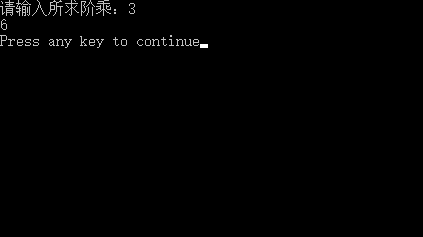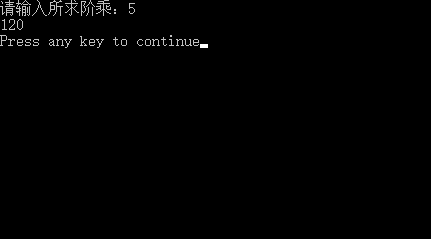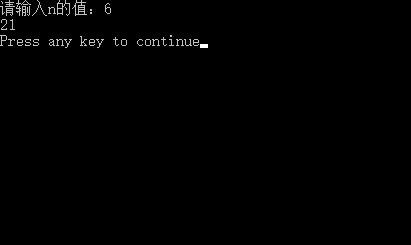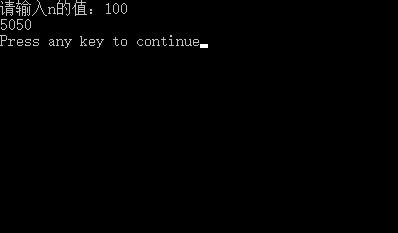• C语言递归函数 1.求阶乘： #include<stdio.h> int recursion(int num) { if(num==1) return 1; else { num=num*recursion(num-1); return num; } ...
C语言递归函数
1.求阶乘：
    #include<stdio.h>

int recursion(int num)

{
if(num==1)
return 1;
else
{
num=num*recursion(num-1);
return num;
}
}

int main()
{
int i;
printf("请输入所求阶乘：");
scanf("%d",&i);
printf("%d\n",recursion(i));
return 0;
}2.求前n项和：
#include<stdio.h>

int recursion(int n)
{
int sum;
if(n==1)
return 1;
else
{

sum=n+recursion(n-1);
return sum;
}
}

int main()
{
int i;
printf("请输入n的值：");
scanf("%d",&i);
printf("%d\n",recursion(i));
return 0;
}展开全文• C语言 递归求阶乘 利用一个函数进行递归求阶乘，但该数不能小于0，且若为0或1，得到的结果都为1，其他情况，对该数不断递归 #include <stdio.h> #include <stdlib.h> int main() { int fac(int n); /...
C语言 递归法求阶乘
利用一个函数进行递归求阶乘，但该数不能小于0，且若为0或1，得到的结果都为1，其他情况，对该数不断递归
#include <stdio.h>
#include <stdlib.h>
int main()
{
int fac(int n);              // 函数声明
int n;             //声明要阶乘的数为int型
int y;             //声明结果为int型
printf("Please input an integer numbers:");
scanf("%d",&n);              // 输入要阶乘的数
y=fac(n);
printf("%d!=%d\n",n,y);
system("pause");
return 0;
}

int fac(int n)                // 定义fac函数
{
int f;
if(n<0)                     // n不能小于0
printf("n<0,data error!");
else if( n==0 || n==1)         // n=0 or n=1 ，阶乘都为1
f=1;
else f=fac(n-1)*n;           // n>1时，n!=n*(n-1)
return(f);
}
//递归法求阶乘



展开全文• C语言练习程序，采用递归方法求阶乘.调用子函数实现
• 递归函数求阶乘 int jie(int n) { if(n<=0) return 1; else{return n*jie(n-1); } }
递归函数求阶乘

int jie(int n)
{
if(n<=0)
return 1;
else{return n*jie(n-1);
}
}

展开全文• 递归函数的定义： 先引入一个例子：输入n，n！。 分析： n！= 1 * 2 * 3 * … * n 1)一般解法： #include"stdio.h" int main() { int n, i; double s = 0; scanf("%d", &n); for(i = 1;i <= n;i++) { ...
递归函数的定义：一个函数在它的函数体内调用它自身称为递归调用，这种函数称为递归函数。递归函数就是反复调用其自己。递归函数要有两要素：1.递归表达式 2.终止条件
先引入一个例子：输入n，求n！。
分析：
n！= 1 * 2 * 3 * … * n
1)一般解法：
#include"stdio.h"
int main()
{
int n, i;
double s = 0;
scanf("%d", &n);
for(i = 1;i <= n;i++)
{
s *= i;
}
printf("%lf", s);
return 0;
}
先分析一下5！：
5！=1 * 2 * 3 * 4 * 5
||
5！=5 * 4 * 3 * 2 * 1
||
5！= 5 * 4！		如果要计算5！先要计算出4！
4！= 4 * 3！		如果要计算4！先要计算出3！
3！= 3 * 2！		如果要计算3！先要计算出2！
2！= 2 * 1！		如果要计算2！先要计算出1！
1！=1			1！就是1
上面从上至下的分析过程称之为递推
上述分析发现我们并没有计算5！的答案，那怎样算出5！的答案？
把分析的过程倒过来：
把1！的结果1代入上一个表达式中执行2 * 1，得到2！的结果2
把2！的结果2代入上一个表达式中执行3 * 2 ，得到3！的结果6
把3！的结果6代入上一个表达式中执行4 * 6，得到4！的结果24
把4！的结果24代入上一个表达式中执行5 * 24，得到5！的结果120
这个过程我们称之为递归
递归算法是一个基础类的算法，也是一个比较容易理解的算法，但是递归的扩展能力是无限的。
我们把上述分析变换成编程语言：
fact(5)=5 * fact(4)
fact(4)=4 * fact(3)
fact(3)=3 * fact(2)
fact(2)=2 * fact(1)
fact(1)=1
归纳出来就是：
fact(n)=n*fact(n-1)		如果要执行该操作必须满足条件（递归算法表达式）
fact(1)=1				如果要执行该操作必须满足条件（终结条件）
2)解法：
#include"stdio.h"
double fact(double n)
{
double s;
if(n >= 2)
{
s = n*fact(n-1);
}
else if(n ==1)
{
s = 1;
}
return s;//不输出因为每次计算的fact结果是用作其他数值的计算所用，另做他用，需要返回值。

}
int main()
{
double s,n;
scanf("%lf", &n);
s = fact(n);
printf("%lf",s);
return 0;
}


展开全文算法 编程语言
• 自己学习C语言已经三年了，学习其实...c语言学习---------递归函数求阶乘 代码： #include int jiec(int num){  if(num  if(num==0) return 1;  return num*jiec(num-1);   } int main(int argc,char *
• ## c语言递归求阶乘和

千次阅读 2020-03-17 17:52:32
本题要求实现一个计算非负整数阶乘的简单函数，并利用该函数求 1!+2!+3!+…+n! 的值。 函数接口定义： double fact( int n ); double factsum( int n ); 函数fact应返回n的阶乘，建议用递归实现。函数factsum应返回...
• 6-9 递归求阶乘和 (15 分) 本题要求实现一个计算非负整数阶乘的简单函数，并利用该函数求 1!+2!+3!+…+n! 的值。 函数接口定义： double fact( int n ); double factsum( int n ); 函数fact应返回n的阶乘，建议用...程序设计
• 1.将递归求阶乘封装函数 2.求1！-10！的和 代码实现： #include<stdio.h> int jiecheng(int n){ if(n==0){ //递归正常结束 return 1; } return n*jiecheng(n-1);//递归调用 } int main(){ int n=0; ...
• ## C语言递归函数练习

千次阅读 2020-05-18 17:58:24
利用递归函数求阶乘 本题要求实现1个自定义函数：求阶乘函数 函数接口定义： long long fac(int); 裁判测试程序样例： 输入 输入正整数n(n<=20) 测试数据有多组，处理到输入结束 输出 每行一个输出结果。 样例...
• 3.递归函数解决问题的典型就是：求阶乘、求斐波那契数列。（这个面试常有要练习一下）。 函数的递归调用原理 1.实际上递归函数是在栈内存上递归执行的，每次递归执行一次就耗费一些栈内存。 2.栈内存的大小是限制...
• 首先我们都知道代码的执行是从上到下的从左到右，函数在运行到递归处就会再次进入自己，只是这次额参数改变了，这里需要注意的事递归函数必须要要一个出口 先上代码： #include<stdio.h> int fun(int n); int ...嵌入式Linux
• //函数调用+递归法 /* ========================================================== 题目：任意整数的阶乘(n ========================================================== */ #include double J(long n) ...
• /* * C语言 数字的阶乘 */ #include #include <stdlib.h>long jiecheng(int n);void main() { int n=0; printf("input a integer number!\n"); scanf("%d",&n); if(n) { printf(
• fun函数使用递归，每次调用自己，阶乘。 #include<stdio.h> int fun(int n) { if(n==1) return 1; else return fun(n-1)*n;//例如5的阶乘就等于(4的阶乘)*5,4的阶乘等于(3的阶乘)*3··· } int ...
• 思路：在子函数中做5的阶乘并使用递归自我调用，在主函数中输出每一次计算的结果。 #include<stdio.h> #include<Windows.h> #include<string.h> int fact(int j) { int sum; if (j == 1) { ...
• //递归函数 f int f(int a) { if(a<=1) return 1; else return a*f(a-1); //实现递归 } int main() { int n,result; scanf("%d",&n); result = f(n); printf("%d",result); r...算法
• 2019独角兽企业重金招聘Python工程师标准>>> ...
• 递归实现n的阶乘 什么是阶乘：0！= 1，n！=n * (n - 1) * (n - 2)......3 * 2 * 1； 解题思路：1>... 很好看出，递归调用自己，直到n等于0，返回之前的函数，直到最后一个；3> 一个简单n的阶乘就计算完...
• 求阶乘函数： int factorial(int number)//自定义阶乘函数 { int temp;//定义整型变量 if(number<0)//如果这个数小于0 { printf("错误数据请，输入大于0的数！");//不符合条件，无法求 } else if...算法
• 例1：使用递归函数求斐波那契数列 斐波那契数列可表示为：F(1)=1，F(2)=1, F(n)=F(n - 1)+F(n - 2)（n ≥ 3，n ∈ N*） 求数列第n项可以用递归函数，只有当n1和n2时函数结束；其他情况一直调用他本身。 代码： #...
• 小例子:n的阶乘！ #include&amp;lt;stdio.h&amp;gt; int fun(int a) { if(a&amp;lt;=1) return 1; return(a*fun(a-1)); } int main() { int a,b; printf(&quot;input a num:\...
• 题目: 利用递归方法实现一个函数,该函数能够实现n的阶乘,即 n! = n*(n-1)*…*3*2*1; #include int factorial(int n) { if(n == 1) //结束递归判断条件 { return; } return n*factorial(n - 1); //递归调用...
• C语言程序举例1、1 到 n 的累加2、递归求阶乘3、递归指数函数4、交集密集函数5、计算Ack函数6、斐波拉契数列7、十进制转二进制8、按顺序输出整数 1、1 到 n 的累加 #include<stdio.h> void main() { int i; ...c++ 算法 动态规划 程序设计
• 就我的理解，就是将递归函数多加了几个参数，并将结果保存在参数中，这就略去了函数回调的代价。以往我们斐波那契(假设从0开始，0，1，1，2......)，基本就是按照递归式子来：if (n == 1) return 0; else if (n...斐波那契
• c语言 递归函数（斐波那契数、n^k、整数各个位数之和、字符反向排列、n的阶乘、打印整数每一位） 1.递归和非递归分别实现第n个斐波那契数。 递归的方法 #define _CRT_SECURE_NO_WARNINGS #include <stdio.h>...
• 输入整型n（n>=0），输出n! #include int fac(int n) { int sum; if(n==1||n==0) sum=1; else sum=n*fac(n-1); return sum; } int main() { int n; scanf("%d",&n); printf("%d",fac(n))...

# c语言递归函数求阶乘c语言 订阅